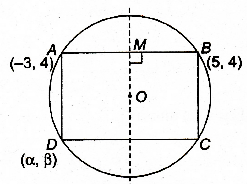21 views

One of the diameter of the circle circumscribing the rectangle ABCD is 4y = x + 7. If A and B are the points (-3, 4) and (5, 4) respectively, then find the area of rectangle

| 21 views

Let O be the centre of circle M be mid point of AB.Then , OM $$\perp$$ AB $$\Rightarrow$$ M (1,4)

Since, slope of AB = 0

Equation of straight line MO is x = 1 and equation of diameter is $$4y = x + 7$$.

$$\Rightarrow$$ Centre is (1, 2).

Also, O is mid point of BD

$$\Rightarrow(\frac{\alpha+5}{2},\frac{\beta+4}{2})=(1,2)$$

$$\Rightarrow\alpha =-3,\beta =0$$

$$\therefore AD=\sqrt{(-3+3)^2+(4-0)^2}=4$$

and $$AB=\sqrt{64+0}=8$$

Thus, area of rectangle is $$8\times4=32\;sq\;unit$$

by (10.3k points)